# Tables

Master Excel tables and analyze your data quickly and easily. Learn how to create a table, sort a table, filter a table, and much more.

### Create a Table

To create a table, execute the following steps.

1. Click any single cell inside the data set.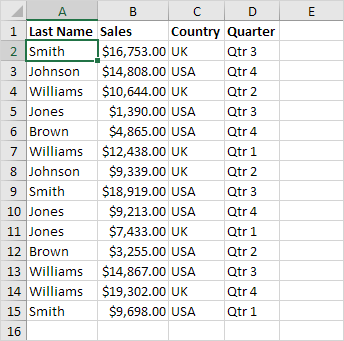2. On the Insert tab, in the Tables group, click Table (or simply press Ctrl + T).The Create Table dialog box appears.

3. Excel automatically selects the data for you. Check 'My table has headers' and click on OK.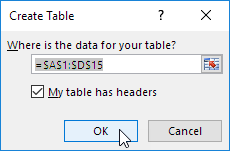Result. Excel creates a nicely formatted table for you. This may still seem like a normal data range to you but many powerful features are now just a click of a button away.### Sort

To sort by Last Name first and Sales second, execute the following steps.

1. First, click the arrow next to Sales and click Sort Smallest to Largest.

2. Next, click the arrow next to Last Name and click Sort A to Z.

Result.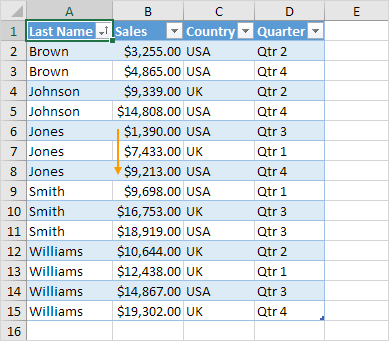### Filter

To filter a table, execute the following steps.

1. Click the arrow next to Country and only check USA.

Result.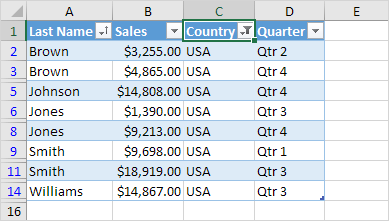### Total Row

To display a total row at the end of the table, execute the following steps.

1. First, select a cell inside the table. Next, on the Table Design tab, in the Table Style Options group, check Total Row (or simply press Ctrl + Shift + T).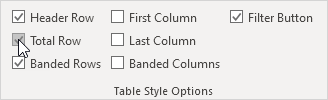Result.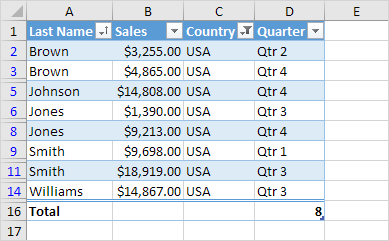2. Click any cell in the last row to calculate the Total (Average, Count, Max, Min, Sum etc.) of a column. For example, calculate the sum of the Sales column.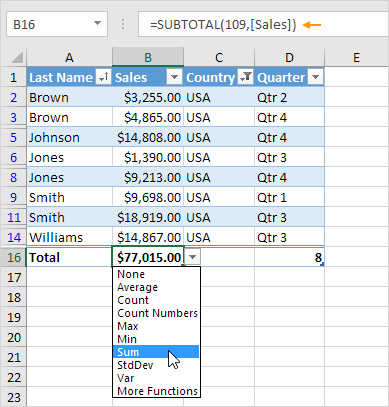Note: in the formula bar, see how Excel uses the SUBTOTAL function to calculate the sum. 109 is the argument for Sum if you use the SUBTOTAL function. Excel uses this function (and not the standard SUM function) to correctly calculate table totals of filtered tables.

### Table Name

Each table has a unique name. You can use this name in formulas. If you like, you can edit the name of a table.

1. First, select a cell inside the table. Next, on the Table Design tab, in the Properties group, we can see that Table1 is the name of this table.2. The formula below refers to Table1 and counts the total number of records.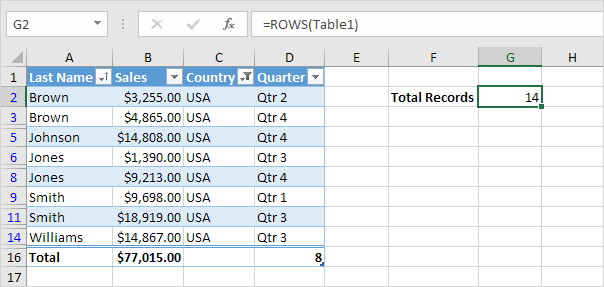### AutoExpansion

Tables in Excel expand automatically when adding new rows or columns. This is a great feature.

1. First, select a cell inside the table.

2. Press Ctrl + Shift + T to quickly remove the total row.

3. Select cell A16 and type Brown (Excel automatically formats this new row for you).Conclusion: this new row is automatically part of the table. All formulas referring to Table1 will be updated automatically (see cell G2). Wow!

Go to Next Chapter: What-If Analysis